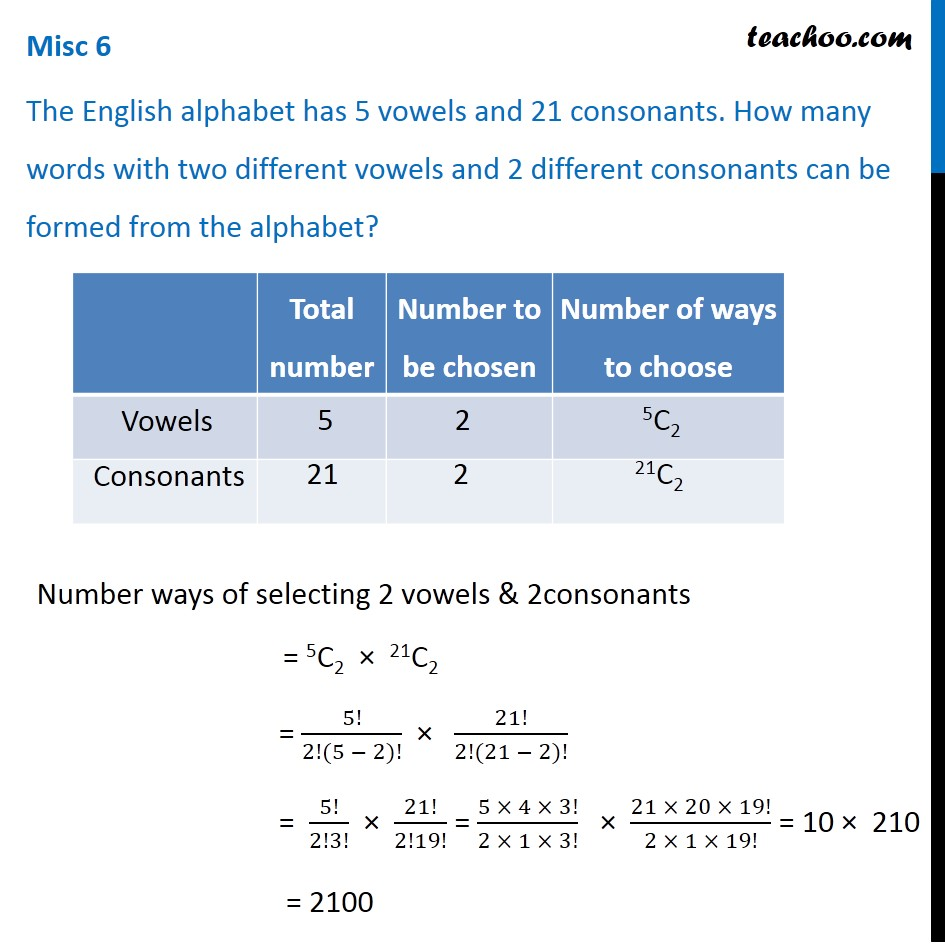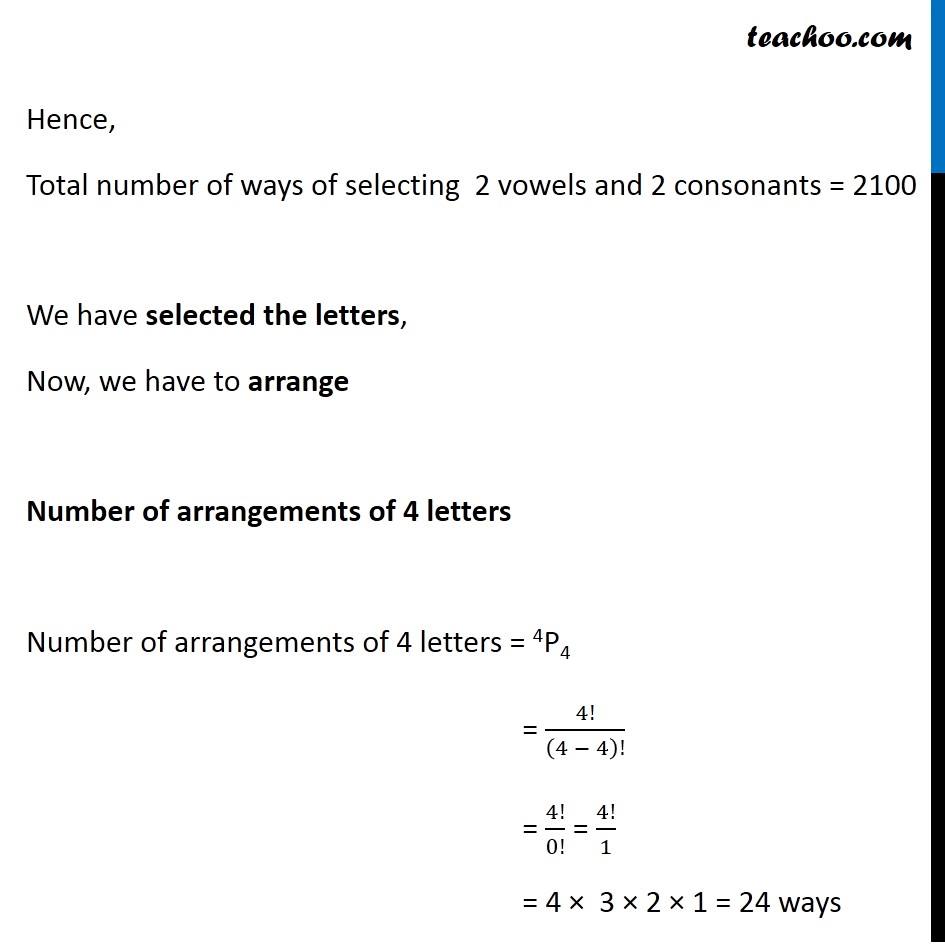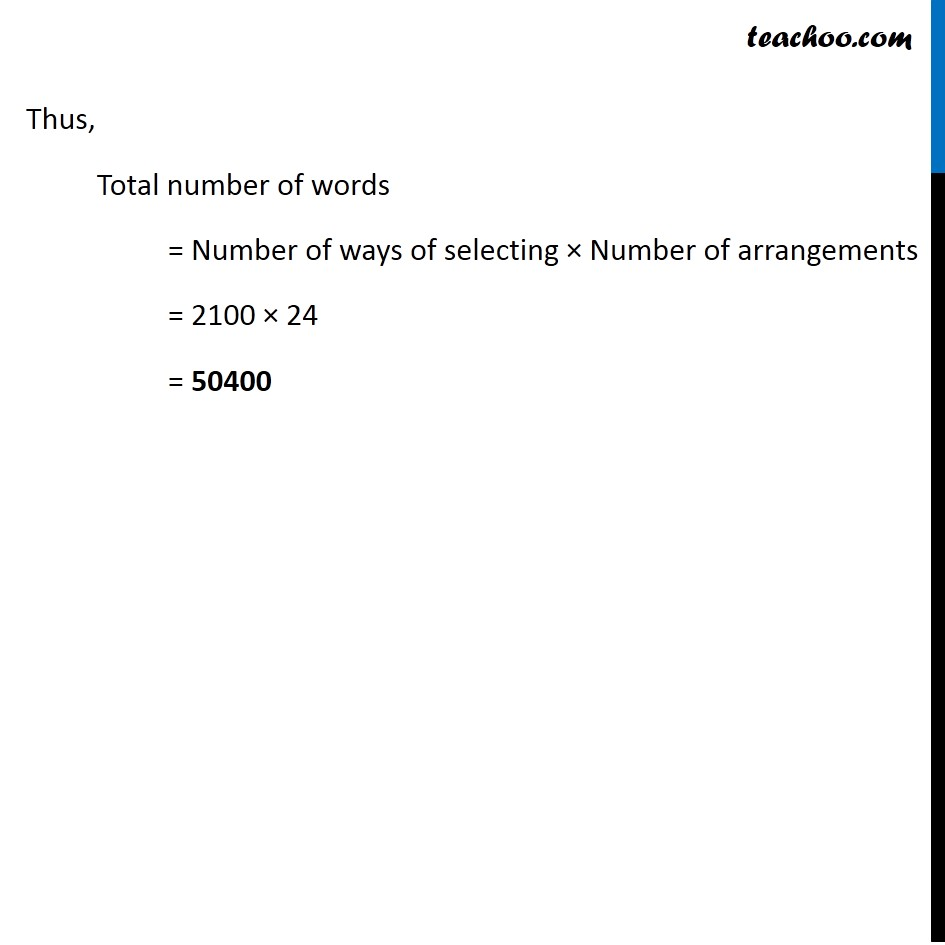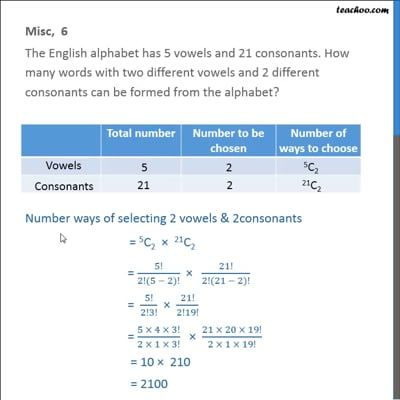Miscellaneous

Chapter 7 Class 11 Permutations and Combinations (Term 2)
Serial order wiseThis video is only available for Teachoo black users

### Transcript

Misc 6 The English alphabet has 5 vowels and 21 consonants. How many words with two different vowels and 2 different consonants can be formed from the alphabet? Number ways of selecting 2 vowels & 2consonants = 5C2 × 21C2 = 5!/(2!(5 − 2)!) × 21!/(2!(21 − 2)!) = 5!/2!3! × 21!/2!19! = (5 × 4 × 3!)/(2 × 1 × 3!) × (21 × 20 × 19!)/(2 × 1 × 19!) = 10 × 210 = 2100 Hence, Total number of ways of selecting 2 vowels and 2 consonants = 2100 We have selected the letters, Now, we have to arrange Number of arrangements of 4 letters Number of arrangements of 4 letters = 4P4 = 4!/(4 − 4)! = 4!/0! = 4!/1 = 4 × 3 × 2 × 1 = 24 ways Thus, Total number of words = Number of ways of selecting × Number of arrangements = 2100 × 24 = 50400